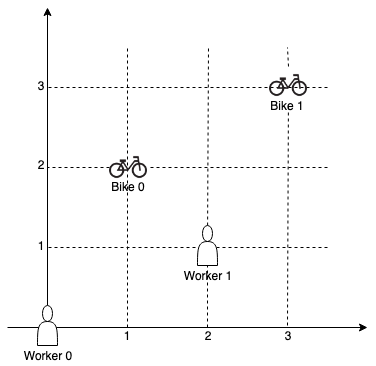1057. Campus Bikes

On a campus represented as a 2D grid, there are `N` workers and `M` bikes, with `N <= M`. Each worker and bike is a 2D coordinate on this grid.

Our goal is to assign a bike to each worker. Among the available bikes and workers, we choose the (worker, bike) pair with the shortest Manhattan distance between each other, and assign the bike to that worker. (If there are multiple (worker, bike) pairs with the same shortest Manhattan distance, we choose the pair with the smallest worker index; if there are multiple ways to do that, we choose the pair with the smallest bike index). We repeat this process until there are no available workers.

The Manhattan distance between two points `p1` and `p2` is `Manhattan(p1, p2) = |p1.x - p2.x| + |p1.y - p2.y|`.

Return a vector `ans` of length `N`, where `ans[i]` is the index (0-indexed) of the bike that the `i`-th worker is assigned to.

Example 1:```Input: workers = [[0,0],[2,1]], bikes = [[1,2],[3,3]]
Output: [1,0]
Explanation:
Worker 1 grabs Bike 0 as they are closest (without ties), and Worker 0 is assigned Bike 1. So the output is [1, 0].
```

Example 2:```Input: workers = [[0,0],[1,1],[2,0]], bikes = [[1,0],[2,2],[2,1]]
Output: [0,2,1]
Explanation:
Worker 0 grabs Bike 0 at first. Worker 1 and Worker 2 share the same distance to Bike 2, thus Worker 1 is assigned to Bike 2, and Worker 2 will take Bike 1. So the output is [0,2,1].
```

Note:

1. `0 <= workers[i][j], bikes[i][j] < 1000`
2. All worker and bike locations are distinct.
3. `1 <= workers.length <= bikes.length <= 1000`

1057. Campus Bikes
``````struct Solution;

use std::cmp::Ordering;
use std::collections::BTreeMap;

struct Point {
x: i32,
y: i32,
}

impl Point {
fn new(v: &[i32]) -> Self {
Point { x: v, y: v }
}
fn manhattan(&self, other: &Point) -> i32 {
(self.x - other.x).abs() + (self.y - other.y).abs()
}
}

#[derive(PartialEq, Eq)]
struct Pair {
i: usize,
j: usize,
}

impl Ord for Pair {
fn cmp(&self, other: &Pair) -> Ordering {
let res = other.i.cmp(&self.i);
if let Ordering::Equal = res {
other.j.cmp(&self.j)
} else {
res
}
}
}

impl PartialOrd for Pair {
fn partial_cmp(&self, other: &Pair) -> Option<Ordering> {
Some(self.cmp(other))
}
}

impl Solution {
fn assign_bikes(workers: Vec<Vec<i32>>, bikes: Vec<Vec<i32>>) -> Vec<i32> {
let mut btm: BTreeMap<i32, Vec<Pair>> = BTreeMap::new();
let n = workers.len();
let mut res: Vec<i32> = vec![0; n];
let m = bikes.len();
for i in 0..n {
for j in 0..m {
let worker = Point::new(&workers[i]);
let bike = Point::new(&bikes[j]);
let distance = worker.manhattan(&bike);
btm.entry(distance).or_default().push(Pair { i, j });
}
}
let mut workers: Vec<bool> = vec![true; n];
let mut bikes: Vec<bool> = vec![true; m];
for (_, mut pairs) in btm.into_iter() {
pairs.sort_unstable();
while let Some(p) = pairs.pop() {
if workers[p.i] && bikes[p.j] {
let i = p.i;
let j = p.j;
workers[i] = false;
bikes[j] = false;
res[i] = j as i32;
}
}
}
res
}
}

#[test]
fn test() {
let workers: Vec<Vec<i32>> = vec_vec_i32![[0, 0], [2, 1]];
let bikes: Vec<Vec<i32>> = vec_vec_i32![[1, 2], [3, 3]];
let res = vec![1, 0];
assert_eq!(Solution::assign_bikes(workers, bikes), res);
let workers: Vec<Vec<i32>> = vec_vec_i32![[0, 0], [1, 1], [2, 0]];
let bikes: Vec<Vec<i32>> = vec_vec_i32![[1, 0], [2, 2], [2, 1]];
let res = vec![0, 2, 1];
assert_eq!(Solution::assign_bikes(workers, bikes), res);
}
``````